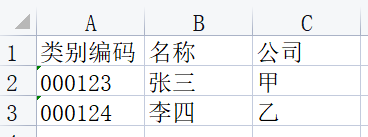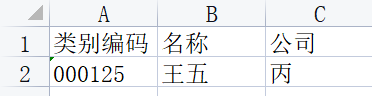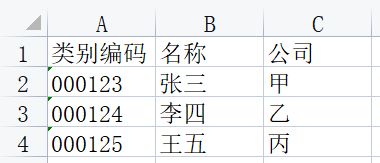• python合并两个表格
2021-09-01 23:26:10

# python 合并多个表格

亲测完毕，开森
版本：python 3.7
xlrd需要安装1.2版本

import xlrd,xlsxwriter,glob
import os

biao_tou = "NULL"
wei_zhi = "NULL"

# 获取要合并的所有excel表格
def get_exce():
global wei_zhi
wei_zhi = input("请输入Excel文件所在的目录：")
all_excel = glob.glob(wei_zhi + "*.xlsx")
print("该目录下有" + str(len(all_excel)) + "个excel文件")
if(len(all_excel) == 0):
return 0
else:
for  i in range(len(all_excel)):
print(all_excel[i])
return all_excel

# 打开Excel文件
def open_excel(name):
fh = xlrd.open_workbook(name)
return fh

# 获取Excel文件下所有Sheet
def get_sheet(fh):
sheets = fh.sheets()
return sheets

# 获取sheet下有多少行数据
def get_sheetrow_num(sheet):
return sheet.nrows

# 获取sheet下的数据
def get_sheet_data(sheet,row):
for i in  range(row):
if (i == 0):
global biao_tou
biao_tou = sheet.row_values(i)
continue
values = sheet.row_values(i)
all_data1.append(values)
return  all_data1

if __name__=='__main__':
all_excel = get_exce()
# 得到要合并的所有excel表格数据
if (all_excel == 0):
print("该目录下无.xlsx文件！请检查您输入的目录是否有误！")
os.system('pause')
exit()

all_data1 = []
# 用于保存合并的所有行的数据

# 下面开始文件数据的获取
for excel in  all_excel:
fh = open_excel(excel)
# 打开文件
sheets = get_sheet(fh)
# 获取文件下sheet的数量

for sheet  in  range(len(sheets)):
row = get_sheetrow_num(sheets[sheet])
# 获取一个sheet下的所有数据的行数

all_data2 = get_sheet_data(sheets[sheet],row)
# 获取一个sheet下的所有行的数据

all_data2.insert(0,biao_tou)
# 表头写入

# 开始文件数据的写入
new_excel = wei_zhi + "test.xlsx"
# 新建的excel文件名字

fh1 = xlsxwriter.Workbook(new_excel)
# 新建一个excel表

# 新建一个sheet表

for i in  range(len(all_data2)):
for j in range(len(all_data2[i])):
c = all_data2[i][j]
net_sheet.write(i,j,c)

fh1.close()

print("文件合并成功请查看" + wei_zhi + "目录下的test.xlsx文件！")

os.system('pause')
os.system('pause')python
更多相关内容
• ## python合并excel表格

千次阅读 2021-12-20 17:02:32
现要将上面两个表格合并如下： 代码： import pandas as pd import os #文件路径 file_dir1 = 'xxx1.xls' file_dir2 = 'xxx2.xls' #构建新的表格名称 new_filename = 'xxx3' new_list = [] dataframe1 = pd.read_...

示例：两个excel表格如下：现要将上面两个表格合并如下：代码：

import pandas as pd
import os

#文件路径
file_dir1 = 'xxx1.xls'
file_dir2 = 'xxx2.xls'
#构建新的表格名称
new_filename = 'xxx3'
new_list = []

#若要保留类型编码列'000123'数据前面的零，使用converters属性
#dataframe1 = pd.read_excel (file_dir1, converters={'类别编码':str})
#保存到新列表中
new_list.append(dataframe1)
#保存到新列表中
new_list.append(dataframe2)
#多个DataFrame合并为一个
df = pd.concat(new_list)
#写入到一个新excel表中
df.to_excel(new_filename,index=False)


展开全文python
• 就是把两个表直接合在一起。于是有一个突出的问题，是横向拼接还是纵向拼接，所以concat 函数的关键参数是axis 。 函数的具体参数是： concat(objs,axis=0,join='outer',join_axes=None,ignore_index=False,keys=...
• python代码，写的合并表格的小内容

## 搜罗出给定路径下的所有文件

• 这里使用了递归遍历
• 还需要注意剔除不是表格的文件
def get_file_path(file_list, path):
try:
if len(file_list) == 0:
# 获取文件夹下是所有文件夹
file_list = os.listdir(path)
if len(file_list) > 0:
for folder in file_list:
if '.' in folder:
str_list = folder.split('.')
if str_list[len(str_list) - 1] in ['xlsx', 'xls']:
# 将表格存入数组
file_path = path + "\\" + folder
file_path_list.append(file_path)
else:
# 深入下一层文件夹
folder_path = path + "\\" + folder
# 获取该文件夹下的所有内容(表格及文件夹)
file_list_one = os.listdir(folder_path)
if len(file_list) > 0:
# 递归调用,筛选出表格
get_file_path(file_list_one, folder_path)
else:
return
except Exception as e:
print("搜罗出现错误,错误原因如下: ", e)
return
print("表格搜罗完成")



## 合并表格内容

• 这里需要将表头不一致的表格进行分类
def merge_excel(path_list, file_path):
if len(path_list) > 0:
df_list = []
title_list = []
try:
for path in path_list:
# 根据表格路径读取表格内容
# 获取表头
titles = df.columns.values
if len(title_list) == 0:
df_son_list = [df]
df_list.append(df_son_list)
title_list.append(titles)
else:
is_same = False
# 遍历表头数组,根据表头对表格进行分类
for index in range(len(title_list)):
title_name_list = title_list[index]
if len(title_name_list) == len(titles):
for i in range(len(title_name_list)):
# 表头不一致
if title_name_list[i] != titles[i]:
# 出现表头不一致的,就跳出循环,继续检查下一个
is_same = False
break
else:
is_same = True
if is_same:
# 将表格数据存入与其表头相同的表格数组
df_list[index].append(df)
break
if not is_same:
# 表头不同的表格, 往末尾添加
df_son_list = [df]
df_list.append(df_son_list)
title_list.append(titles)
except Exception as e:
print("表格分类出错错误,错误原因如下: ", e)
print("错误路径如下: ", path)
try:
if len(df_list) > 0:
print("文件分类完成, 共有{}类, 开始合并: ".format(df_list.__len__()))
for index in range(len(df_list)):
df_list_son = df_list[index]
# 将读取的内容合并
merge_df = pd.concat(df_list_son, axis=0)
# 设置合并后文件的存储路径
new_file_path = file_path + "-合并后\\" + str(index) + "-合并表格.xlsx"
# 生成表格
merge_df.to_excel(new_file_path, index=0)
except Exception as e:
print("表格合并出错,错误原因如下: ", e)
print("大功告成")



## 调用上面的函数

file_path_list = []
# 存放文件的路径,这种是绝对路径,可以自己根据需要修改为任意磁盘的任意位置
# one_path = 'E:\\01-Python\\ManualPractis\\File\\2022-05-24'
# 存放表格的相对路径,一般只能放在项目当中
one_path = '../File/2022-05-24'
get_file_path([], one_path)
merge_excel(file_path_list, one_path)

展开全文python
• 本文实例讲述了Python实现合并excel表格的方法。分享给大家供大家参考，具体如下：需求将一文件夹中的excel表格合并成我们想要的形式，主要要pandas中的concat()函数思路用os库将所需要处理的表格放到同一列表中...

本文实例讲述了Python实现合并excel表格的方法。分享给大家供大家参考，具体如下：

需求

将一个文件夹中的excel表格合并成我们想要的形式，主要要pandas中的concat()函数

思路

用os库将所需要处理的表格放到同一个列表中，然后遍历列表，依次把所有文件纵向连接起来。

最开始的第一种思路是先拿一个文件出来，然后让这个文件依次去和列表中的剩余文件合并；

第二种是用文件夹中第一个文件和剩余的文件合并，使用range(1,len(file))，可以省去单独取第一个文件的步骤。

遇到的问题

读取

好久没写过这个了，竟然在读取的时候出了很多错误，花了很多时间，下面按时间顺序小结一下

#以前的操作方式,需要占用三行

df = pd.read_excel(r'C:.\1.xlsx', sheet_name = '设备检测_空调伴侣')

temp_columns = ['','']

df_1 = df[temp_columns].dropna()

#但我在进阶的过程中，格式弄错了，导致一直报错

df = pd.read_excel(r'C:.\1.xlsx', sheet_name = '设备检测_空调伴侣').[['采集时间']['功率E(W)']]

>>>df = pd.read_excel(r'C:.\1.xlsx', sheet_name = '设备检测_空调伴侣').[['采集时间']['功率E(W)']] ^

SyntaxError: invalid syntax

#发现多了个“.”，用pd读取的excel已经是dataframe的格式了，提取直接用列表的方式就行，加“.”是表示用pd中的函数，完全不同，修正后，再运行，又报错。。。

df = pd.read_excel(r'C:.\1.xlsx', sheet_name = '设备检测_空调伴侣')[['采集时间']['功率E(W)']]

>>>TypeError: list indices must be integers or slices, not str

#发现列表中的子列表元素间忘记用“，”分隔了，修正，再运行

df = pd.read_excel(r'C:.\1.xlsx', sheet_name = '设备检测_空调伴侣')[['采集时间'],['功率E(W)']]

>>>TypeError: unhashable type: 'list'

#说实话，当时心态有点崩，但还是仔细找了找原因，报错，列表是不可拆分的类型。再对比以前的代码，才发现问题所在。

df = pd.read_excel(r'C:.\1.xlsx', sheet_name = '设备检测_空调伴侣')[['采集时间','功率E(W)']]

需要用双层列表，外面那层是用于读取dataframe,里面那层是索引的集合

完整代码

思路1：

# -*- coding: utf-8 -*-

import numpy as np

import pandas as pd

import os

#改变当前的路径

os.chdir(r'C:\Users\hao\Desktop\重写数据整理\源数据')

#将当前目录下的文件以列表的形式存放

file = os.listdir("./")

df_0 = pd.read_excel(r'C:\Users\hao\Desktop\重写数据整理\143NNCZ01_M_2017-06.xlsx', sheet_name = '设备检测_空调伴侣')[['采集时间','功率E(W)']].dropna()

#print(df_0) #df_0是第一个，依次和循环里面的每一个表做纵向连接

for aa,excel in enumerate(file) : #enumerate 遍历，aa 返回序列，便于计数和监视

print('当前正在处理的文件：',excel)

df_1 = pd.read_excel(excel, sheet_name = '设备检测_空调伴侣')[['采集时间','功率E(W)']].dropna()

#print(df_1)

df_0 = pd.concat([df_0,df_1],ignore_index=True, axis=0)

#print(df_0)

df_0.to_excel(r'C:\Users\hao\Desktop\output3.xlsx', index=None)

思路二：

# -*- coding: utf-8 -*-

import numpy as np

import pandas as pd

import os

#改变当前的路径

os.chdir(r'C:\Users\hao\Desktop\重写数据整理\源数据')

#将当前目录下的文件以列表的形式存放

file = os.listdir("./")

df_0 = pd.read_excel(file, sheet_name = '设备检测_空调伴侣')[['采集时间','功率E(W)']].dropna()

#print(df_0) #df_0是第一个，依次和循环里面的每一个表做纵向连接

for i in range(1,len(file)) : #enumerate 遍历，aa 返回序列，便于计数和监视

print('当前正在处理的文件：',file[i],'第{}/{}个'.format(i+1,len(file)+1))

df_1 = pd.read_excel(file[i], sheet_name = '设备检测_空调伴侣')[['采集时间','功率E(W)']].dropna()

#print(df_1)

df_0 = pd.concat([df_0,df_1],ignore_index=True, axis=0)

#print(df_0)

df_0.to_excel(r'C:\Users\hao\Desktop\output5.xlsx', index=None)

更多关于Python相关内容感兴趣的读者可查看本站专题：《Python操作Excel表格技巧总结》、《Python文件与目录操作技巧汇总》、《Python文本文件操作技巧汇总》、《Python数据结构与算法教程》、《Python函数使用技巧总结》、《Python字符串操作技巧汇总》及《Python入门与进阶经典教程》

希望本文所述对大家Python程序设计有所帮助。

展开全文• 利用python中的pandas来实现操作。 实用场景是在直播时统计参与线上学习的员工的编号及分支机构。用户数据中ID号为唯一。表一：表二： 代码：python
• 问题是有相同列的两个表格合并，但是列中的值顺序不一样，所以需要用到merge函数。 import pandas as pd import os print(os.getcwd()) #打印出当前工作路径 df5 = pd.read_csv("C:\\Users\\hanhan\\...python
• pandas提供concat函数对两个或多个csv文件进行合并。 1.行合并 f1 = pd.read_csv('file1.csv') f2 = pd.read_csv('file2.csv') file = [f1,f2] train = pd.concat(file) train.to_csv("file3" + ".csv", index=0, ...python
• Python数据分析】利用Python将多EXCEL表格合并为一EXCEL表格。想获取代码文件，请微信关注微信公众号“闲谈项目管理”，对话框回复“数据分析案例2”即可获得文件下载链接。python
• 工作中有时候会遇到把多具有相同表头的EXCEL表格的内容合并，本例中表头内容如下： 具体方法： 1将需要合并表格提前汇总到一文件夹中； 2使用os.listdir获取文件夹中各表格的名称； 2使用openpyxl循环...python
• 将多个子文件夹下的相同名字的excel文件合并成一excel，可以直接运行。excel见下面链接 https://amaze.blog.csdn.net/article/details/124889377?spm=1001.2014.3001.5502
• 但我遇到的问题是需要至少两个条件符合后进行不全。希望我的表述清楚。现做了个示例图，应该更好理解问题。感谢! <p><img alt="" height="522" src=...python
• python:实现合并两个列表算法(附完整源码)python 数据结构
• 故事提要：有个运营的朋友， 6张excel 表需要合并到一张表， 每张表大约65536 个数据...正好帮个忙，拿来用python练练手~比如下面两个重复的数据： 合并后的结果是:pwk_id :不变， 访问次数: 1+1 = 2 , 操作系统: P...
• 由于项目取数需要，要将两个不同的csv文件合并到一个文件中，并根据公共列（即两个文件中有一个或几个列的数据应该是对应一致的）合并到同一行，具体代码实现如下： import pandas as pd #读取数据 r1= pd.read_...python
• 需要安装pandas，openpyxl这两个第三方类库，否则会报错。 代码实现： # -*- coding: gbk -*- import os import pandas as pd dir = input("请输入待合并文件的路径：\n") #设置工作路径 frames = [] #存放导入的...
• 想把文本 1 和文本 2 ，合并为文本 3 ， 2 数据源， date 是日期，然后另外 2 不同字段，想合并到一张表中，也就是合并成同一 json ，求助，有办法吗文本 1 ：[{'aaaaa': 0, 'date': '12.13'},{'aaaaa': 0, '...
• 使用Python合并表格只要一句代码！ 1 读取表格 使用Python读取数据只要3行，第一行载入pandas工具，第二行读取，第三行显示头部5行内容。 importpandasaspd data1=pd.read_excel('表格1.xlsx') data1.head() ...python
• ## python实现表格合并

千次阅读 2015-03-06 11:05:42
应用场景：有一文件夹存放着表结构相同的大量表格，现将所有表格合并，表头保留 代码实现： import os import os.path import xdrlib ,sys import xlrd import csv rootdir="e:\min5\sh" csvfile=file('...python
• 利用python批量合并excel和word内的表格python 数据挖掘
• 当你有多列名一致的excel文件的时候，你想要把多excel文件合并成一excel文件 Python代码实现 首先导入需要的库 import pandas as pd import os 将所有需要合并的excel放进一单独的文件夹里 定义一函数...python
• 二、merge “合并” df = pd.merge(left, right, on =["ID", "ID"]) # 实战项目中，同一企业的数据依据 year 竖向排列，要改为横向合并。 def merge_year(data): temp = pd.merge(data[data['year'] ==2016], ...
• 1 场景合并word文件到一文件中，用于文件的批量打印。要求：每文件合并时，均从从一新页面开始，文件之间的内容不连在一起。文件的样式不发生改变。2 方案采用python实现，使用python中的python-docx和...
• 原标题：Python实战：合并 Excel 表格 首先是需求一：有 表 A.xlsx 和 表 B.xlsx，想将其纵向合并成同一张表格: 以及需求二：想在 表 C.xlsx 中提取第三列、在 表 D.xlsx 中提取前两列，整合成新的表格： 如果不用...
• ## python合并两列

千次阅读 2020-11-30 09:12:59
作者： lateautumn4lin 来源：云爬虫技术研究笔记aka逆向小学生今天我们的题目是《由一简单的python合并字典问题引发的思考，如何优化我们的代码？ 为什么会有这话题呢？ 起因是今天和一位刚刚面试完python开发...
• 合并，即横向合并 from openpyxl import Workbook,load_workbook wb=Workbook() wb=load_workbook("excel.xlsx") wslist=wb.worksheets ws1=wslist ws2=wslist ws3=wslist Columns=ws1.columns ...python
• 系统功能：一段python脚本用python做出一个脚本对两个表格的数据匹配：第一个表格是目的文件，issue no是按时间顺序排列，第二个表格是源文件里面issue no是乱序，现在需要将第二个excel文件的jira ID匹配到第一个...python django flask
• 问题描述python中用docx库读取word文件，若word文件中包含合并表格表格则通过docx读取显示：file = docx.Document(path)for table in file.tables:for row in table.rows:for cell in row.cells:print(cell.text)...
• 场景：许多excel文件格式和内容的标题都一样，需要将这些表格合并到一起，有5表，每表10行的话，合并后就是50行的数据 方案1：文件放到一目录中遍历所有文件然后进行数据合并 目录 d:/表格存放/ 下存放了5...python 合并排序...